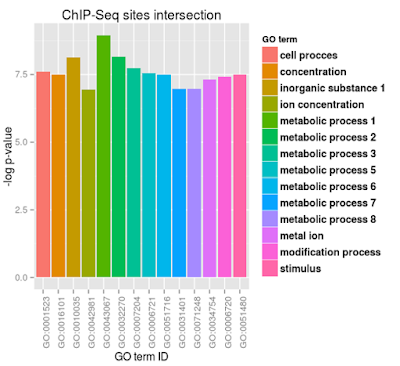## Saturday, March 12, 2016

### Overcome problems with sorting on x-axes and legend in ggplot2

This is one example of R ggplot2 code that will load your data matrix with three columns and make a bar chart with a legend. Data are from GO analysis of ChIP-Seq peaks intersections for two experiments and containg GO term ID, GO name, and p-value.
Now you want to plot -log p-value in a decreasing order, and ggplot2 will not order x-axes by the p-value, but rather in alphabetical order. This is why you would have to specify this your self and it is relatively easy in ggplot2 with setting limits=factor(x\$V2) in scale_x_discrete - where x\$V2 is the vector with categories in order; if data frame is not sorted by the p-value this vector would not make sense so first sort data frame with:
`x<-x[order (x\$value, decreasing=TRUE),]`
and breaks = x\$V2 in scale_fill_discrete for the legend .

```> library("ggplot2")
> library("reshape2")

> x
V1                    V2       V3
1  GO:0001523   metabolic process 1 0.000129
2  GO:0016101   metabolic process 2 0.000287
3  GO:0010035 inorganic substance 1 0.000293
4  GO:0042981   metabolic process 3 0.000433
5  GO:0043067          cell procces 0.000504
6  GO:0032270   metabolic process 5 0.000520
7  GO:0007204         concentration 0.000548
8  GO:0006721   metabolic process 6 0.000550
9  GO:0051716              stimulus 0.000554
10 GO:0031401  modification process 0.000597
11 GO:0071248             metal ion 0.000673
12 GO:0034754   metabolic process 7 0.000947
13 GO:0006720   metabolic process 8 0.000947
14 GO:0051480     ion concentration 0.000963
> x\$V3=-log(x\$V3)
> x<-melt(x)
Using V1, V2 as id variables
> x
V1                    V2 variable    value
1  GO:0001523   metabolic process 1       V3 8.955698
2  GO:0016101   metabolic process 2       V3 8.156028
3  GO:0010035 inorganic substance 1       V3 8.135338
4  GO:0042981   metabolic process 3       V3 7.744773
5  GO:0043067          cell procces       V3 7.592934
6  GO:0032270   metabolic process 5       V3 7.561682
7  GO:0007204         concentration       V3 7.509235
8  GO:0006721   metabolic process 6       V3 7.505592
9  GO:0051716              stimulus       V3 7.498346
10 GO:0031401  modification process       V3 7.423593
11 GO:0071248             metal ion       V3 7.303765
12 GO:0034754   metabolic process 7       V3 6.962211
13 GO:0006720   metabolic process 8       V3 6.962211
14 GO:0051480     ion concentration       V3 6.945457
>  ggplot(x, aes(V2,value, fill = V2)) + geom_bar(stat="identity")+ scale_x_discrete(labels=x\$V1, limits=factor(x\$V2))+ scale_fill_discrete(breaks = x\$V2, name="GO term")+theme(legend.text = element_text(colour="black", size = 11, face = "bold"))+ theme(axis.text.x = element_text(angle = 90, hjust = 1))+ggtitle("ChIP-Seq sites intersection")+labs(x="GO term ID",y="-log p-value")
```
The resulting figure looks like this:

If you did not correct this the graph would not be sorted.

`>  ggplot(x, aes(V2,value, fill = V2)) + geom_bar(stat="identity")+ scale_x_discrete(labels=x\$V1)+ scale_fill_discrete(name="GO term")+theme(legend.text = element_text(colour="black", size = 11, face = "bold"))+ theme(axis.text.x = element_text(angle = 90, hjust = 1))+ggtitle("ChIP-Seq sites intersection")+labs(x="GO term ID",y="-log p-value")`